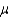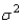# [Solved] Random Samples of Size N Were Selected from Populations with the Means

Question 130

## Random samples of size n were selected from populations with the means and variances given here. Find the mean and standard deviation of the sampling distribution of the sample mean in each case:a. n = 36,= 12,= 9Mean: ______________SD: ______________ b. n = 100,= 15,= 4Mean: ______________SD: ______________ c. n = 8,= 100,= 1Mean: ______________SD: ______________

10+ million students use Quizplus to study and prepare for their homework, quizzes and exams through 20m+ questions in 300k quizzes.

### Statistics

Explore our library and get Introductory Statistics Homework Help with various study sets and a huge amount of quizzes and questions

227

Study sets

3.8K

Quizzes

319.4K

Questions

Upload material to get free accessInvite a friend and get free accessSubscribe and get an instant access